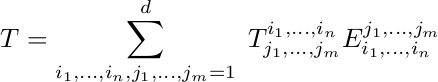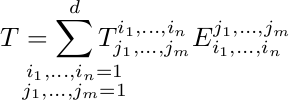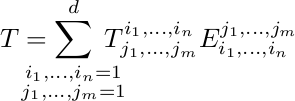In my Differential geometry course there is a section on tensor products and they can get quite messy. Notation-wise that is. One of our professors is convinced the current generation of students is unable to grasp tensor products due to some intoxication in the water or something similar, but here I am speaking only about notation :).

An example, the notes contain a formula depicting the expression of a tensor in a certain basis

producesObviously, the enormous width of the summation indices produces an ugly result. \mathclap and \substack to the rescue!

First, \substack. The relevant part of the manual states

The \substack command can be used to produce a multiline subscript or superscript.

Sounds like what we need, don't you think? We just split up the summation indices in a row of i's and a row of j's.

Now, \mathclap. The relevant part of the mathtools manual states

[...] contains a lot of excessive white space. The idea that comes to mind is to fake the width of the subscript. The command \mathclap puts its argument in a zero width box and centers it, [...]

Aha, LaTeX will ignore the width of the summation indices when typesetting math. That's what we need.

So we get

which producesWhen I look at this rendering of the formula, one thing still annoys me. There is not enough space now between the summation symbol and the summands. I feel like there's some symmetry needed: the space between the equals sign and the summation symbol should be on par with the space between the summation symbol and the summands.

Let's add some hardcoded whitespace, \; is one of the lesser known (at least to me) spacings, representing 5/18th of a quad. That's neither too wide nor thin, so using

we getIn case you're wondering what the name \mathclap means, it helps when you know there is a \mathllap and \mathrlap. So \mathclap centers the zero width box, while the other two respectively align the box to the left and right.